# GMAT Math : DSQ: Calculating the area of a square

## Example Questions

### Example Question #171 : Data Sufficiency Questions

Data sufficiency question- do not actually solve the question

Find the area of a square.

1. The length of one side of the square is 4.

2. The length of the diagonal of the square is 12.

Statement 2 alone is sufficient, but statement 1 alone is not sufficient to answer the question

Statements 1 and 2 together are not sufficient, and additional data is needed to answer the question

Both statements taken together are sufficient to answer the question, but neither statement alone is sufficient

Each statement alone is sufficient

Statement 1 alone is sufficient, but statement 2 alone is not sufficient to answer the question

Each statement alone is sufficient

Explanation:

Because all 4 sides of a square are equal, knowing the length of one side is sufficient to answer the question. Using the Pythagorean Theorem, you can calculate the length of 1 side of a square by knowing the length of a diagonal and then calculate the area.

### Example Question #172 : Data Sufficiency Questions

A circle is inscribed inside Square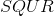. The circle intersects the square at points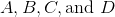. Give the area of the square.

Statement 1: The circle has area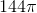.

Statement 2: Arc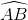has length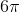.

Statement 2 ALONE is sufficient to answer the question, but Statement 1 ALONE is NOT sufficient to answer the question.

BOTH statements TOGETHER are insufficient to answer the question.

EITHER statement ALONE is sufficient to answer the question.

Statement 1 ALONE is sufficient to answer the question, but Statement 2 ALONE is NOT sufficient to answer the question.

BOTH statements TOGETHER are sufficient to answer the question, but NEITHER statement ALONE is sufficient to answer the question.

EITHER statement ALONE is sufficient to answer the question.

Explanation:

From Statement 1, the radius of the circle can be calculated by working backwards from the area formula, and the diameter can be calculated by doubling this.

From Statement 2, since, by the regularity of the square,is one fourth of the circle, the length ofcan be multiplied by four to get the circumference of the circle. This can be divided byto obtain this diameter.

This diameter is equal to the the sidelength of the square in which it is inscribed, so it can be squared to obtain the area of the square. This makes each statement alone sufficient to answer the question.

### Example Question #173 : Data Sufficiency Questions

A circle is inscribed inside Square. The circle intersects the square at points. Give the area of the square.

Statement 1: The circle has circumference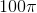.

Statement 2:is aarc.

EITHER statement ALONE is sufficient to answer the question.

BOTH statements TOGETHER are insufficient to answer the question.

Statement 2 ALONE is sufficient to answer the question, but Statement 1 ALONE is NOT sufficient to answer the question.

BOTH statements TOGETHER are sufficient to answer the question, but NEITHER statement ALONE is sufficient to answer the question.

Statement 1 ALONE is sufficient to answer the question, but Statement 2 ALONE is NOT sufficient to answer the question.

Statement 1 ALONE is sufficient to answer the question, but Statement 2 ALONE is NOT sufficient to answer the question.

Explanation:

The diameter of the inscribed circle is equal to the the sidelength of the square. From Statement 1, the circumference can be divided byto obtain this measure, and this can be squared to obtain the area of the square.

Statement 2 gives extraneous information, as, by regularity of the figure, it is already known thatis one fourth of the circle and, subsequently, aarc.

### Example Question #174 : Data Sufficiency Questions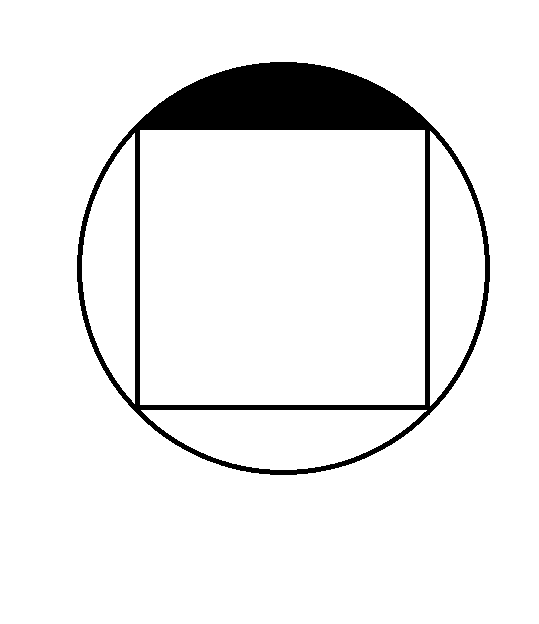The above figure shows a square inscribed inside a circle. What is the area of the black region?

Statement 1: The square has perimeter 40.

Statement 2: The circle has area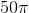.

BOTH statements TOGETHER are insufficient to answer the question.

Statement 2 ALONE is sufficient to answer the question, but Statement 1 ALONE is NOT sufficient to answer the question.

EITHER statement ALONE is sufficient to answer the question.

BOTH statements TOGETHER are sufficient to answer the question, but NEITHER statement ALONE is sufficient to answer the question.

Statement 1 ALONE is sufficient to answer the question, but Statement 2 ALONE is NOT sufficient to answer the question.

EITHER statement ALONE is sufficient to answer the question.

Explanation:

The area of the black region is one-fourth the difference of the areas of the circle and the square.

Ifis the sidelength of the square, then the length of its diagonal - which is also the diameter of the circle - is, by the Pythagorean Theorem,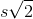, and the radius. Therefore, if you calculate either the radius or the sidelength, you can calculate the other, allowing you to find the areas of the circle and the square.

Statement 1 allows you to find the sidelength; just divide 40 by 4.

Statement 2 allows you to find the radius; just solve forin the equation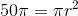.

Therefore, either one gives you enough information to solve the problem.

### Example Question #175 : Data Sufficiency Questions

Find the area square R

I) The diagonal of square R is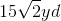.

II) The perimeter of square R is.

Statement 1 is sufficient to solve the question, but statement 2 is not sufficient to solve the question.

Statement 2 is sufficient to solve the question, but statement 1 is not sufficient to solve the question.

Neither statement is sufficient to solve the question. More information is needed.

Each statement alone is enough to solve the question.

Both statements taken together are sufficient to solve the question.

Each statement alone is enough to solve the question.

Explanation:

You can use the diagonal of a square to find its side length via the ratio of a 45/45/90 triangle.

In this case our triangle will have side lengths of 15.

We can also divide our perimeter by 4 to get our side length, which is again 15.

Therefore, each statement alone is sufficient to solve the question.

### Example Question #176 : Data Sufficiency Questions

True or false: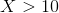Statement 1: A square with sidelengthhas perimeter greater than 100.

Statement 2: A square with sidelengthhas area greater than 40.

STATEMENT 1 ALONE provides sufficient information to answer the question, but STATEMENT 2 ALONE does NOT provide sufficient information to answer the question.

EITHER STATEMENT ALONE provides sufficient information to answer the question.

BOTH STATEMENTS TOGETHER do NOT provide sufficient information to answer the question.

STATEMENT 2 ALONE provides sufficient information to answer the question, but STATEMENT 1 ALONE does NOT provide sufficient information to answer the question.

BOTH STATEMENTS TOGETHER provide sufficient information to answer the question, but NEITHER STATEMENT ALONE provides sufficient information to answer the question.

STATEMENT 1 ALONE provides sufficient information to answer the question, but STATEMENT 2 ALONE does NOT provide sufficient information to answer the question.

Explanation:

Assume Statement 1 alone. The perimeter of a square is four times the length of a side, which here is; the perimeter is greater than 100, so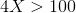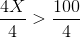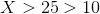Therefore, the question can be answered in the affirmative from Statement 1 alone.

Statement 2 alone gives insufficient information, however. The area of a square is the square of the length of a side. If the sidelength is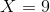, for example, the area is the square of this, which is 81; if the sidelength is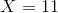, the area is 121. Both areas exceed 40, but only in the second case is.

Tired of practice problems?

Try live online GMAT prep today.